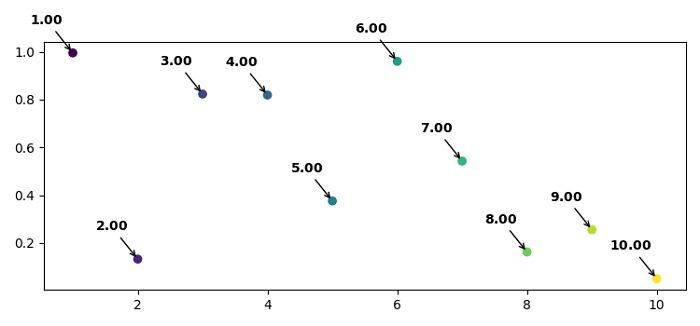# How to add bold annotated text in Matplotlib?

To add bold annotated text in matplotlib, we can use LaTeX representation for labels.

## Steps

• Set the figure size and adjust the padding between and around the subplots.

• Create x and y data points using numpy.

• To set the label for each scattered point, make a list of labels.

• Plot xpoints, ypoints using scatter() method. For color, use xpoints.

• Iterate zipped labels, xpoints and ypoints.

• Use annotate() method with bold LaTeX representation insie the for loop.

• To display the figure, use show() method.

## Example

import numpy as np
from matplotlib import pyplot as plt
plt.rcParams["figure.figsize"] = [7.50, 3.50]
plt.rcParams["figure.autolayout"] = True
xpoints = np.linspace(1, 10, 10)
ypoints = np.random.rand(10)
labels = ["%.2f" % i for i in xpoints]
plt.scatter(xpoints, ypoints, c=xpoints)
for label, x, y in zip(labels, xpoints, ypoints):
plt.annotate(
f"$\bf{label}$",
xy=(x, y), xytext=(-20, 20),
textcoords='offset points', ha='center', va='bottom',
plt.show()